Math Triumphs

Math Triumphs is intended for use as a pull out intervention class (RtI level 3) for students who are struggling in mathematics concepts two or more grade levels below their current grade. It is designed for use in after school, before school, summer school, intercession, tutoring, or pull-out/resource rooms.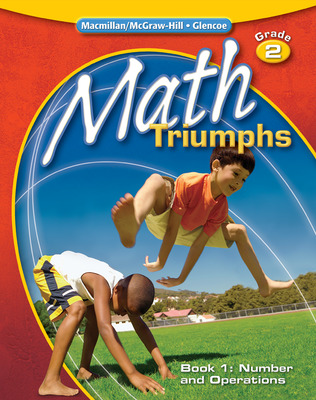Math Triumphs is the first intervention program designed around the NCTM Focal Points. The Student Editions are 4-color, consumable Student Study Guide worktexts focusing on the foundational skills and concepts leading up to one of the three grade level Focal Points. Each chapter begins with a diagnostic assessment to identify concepts and skills that students may need to review or reinforce before introducing the new chapter.

Program Components

•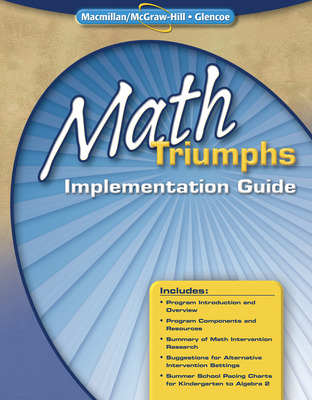Math Triumphs Implementation Guide
•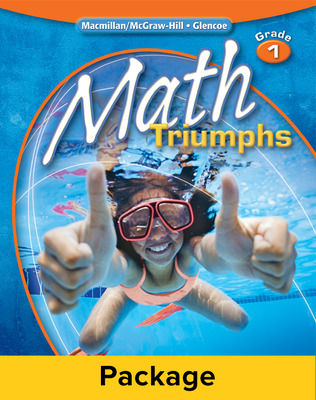Math Triumphs, Grade 1, Classroom Intervention Kit
•Math Triumphs, Grade 1, Teacher Resource Package
•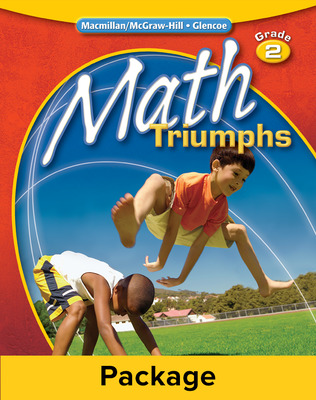Math Triumphs, Grade 2, Classroom Intervention Kit
•Math Triumphs, Grade 2, Teacher Resource Package
•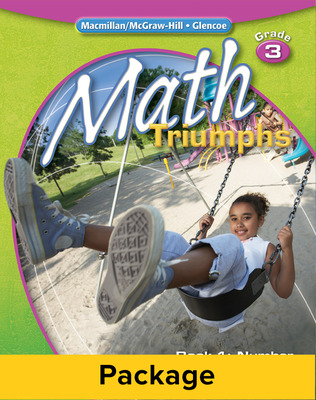Math Triumphs, Grade 3, Classroom Intervention Kit
•Math Triumphs, Grade 3, Teacher Resource Package
•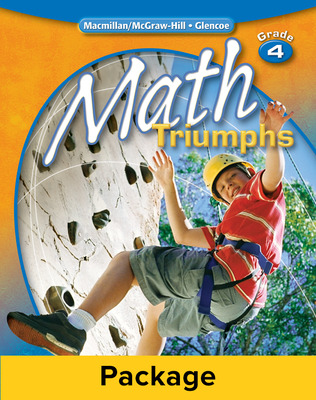Math Triumphs, Grade 4, Classroom Intervention Kit
•Math Triumphs, Grade 4, Teacher Resource Package
•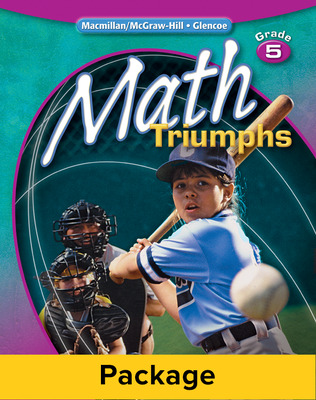Math Triumphs, Grade 5, Classroom Intervention Kit
•Math Triumphs, Grade 5, Teacher Resource Package
•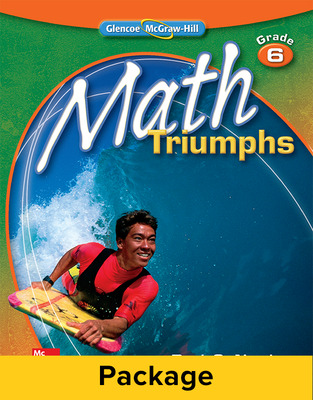Math Triumphs, Grade 6, Classroom Intervention Kit
•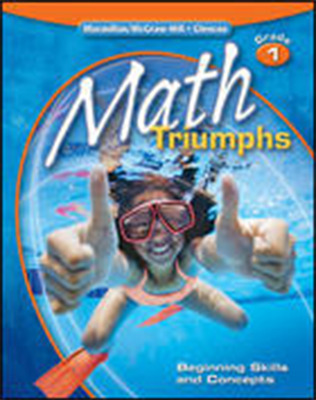Math Triumphs, Grade 1, Assessment Masters
•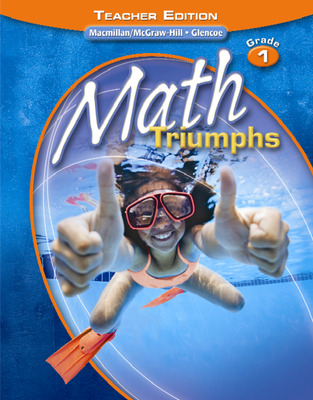Math Triumphs, Grade 1, Teacher Edition
•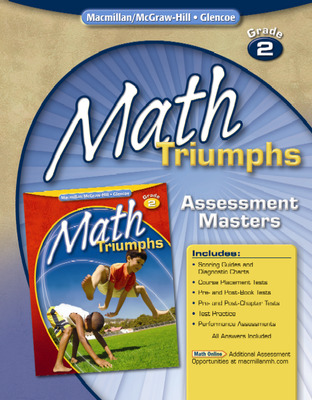Math Triumphs, Grade 2, Assessment Masters
•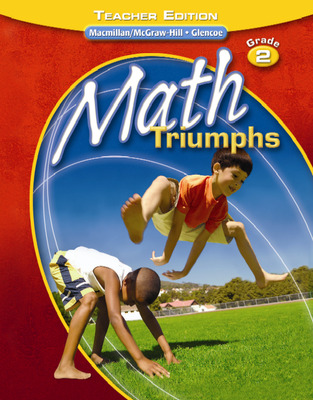Math Triumphs, Grade 2, Teacher Edition
•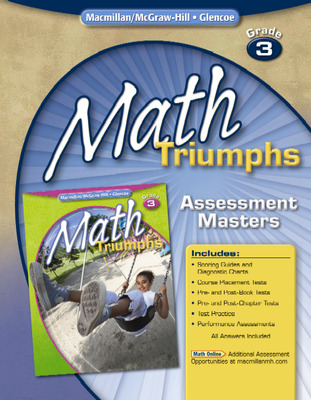Math Triumphs, Grade 3, Assessment Masters
•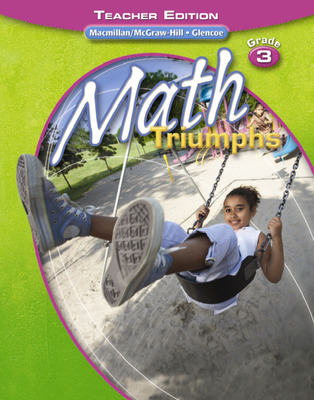Math Triumphs, Grade 3, Teacher Edition
•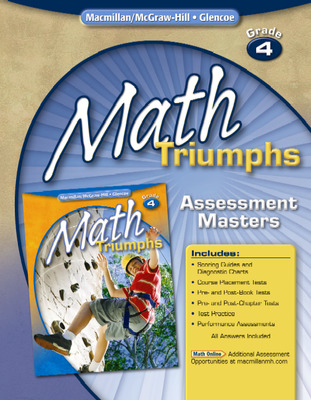Math Triumphs, Grade 4, Assessment Masters
•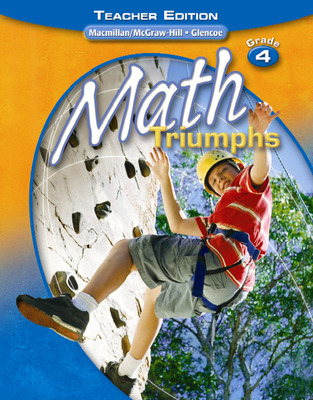Math Triumphs, Grade 4, Teacher Edition
•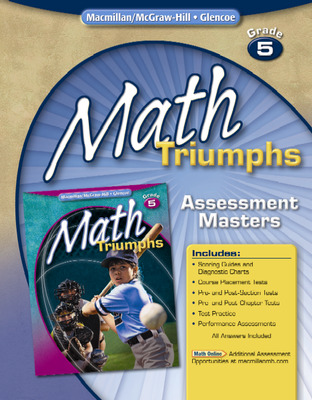Math Triumphs, Grade 5, Assessment Masters
•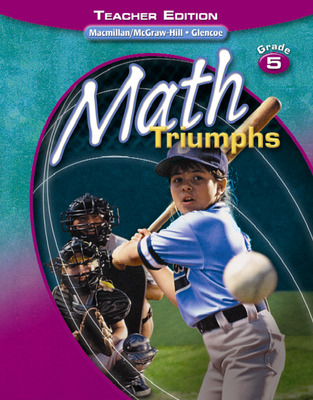Math Triumphs, Grade 5, Teacher Edition
•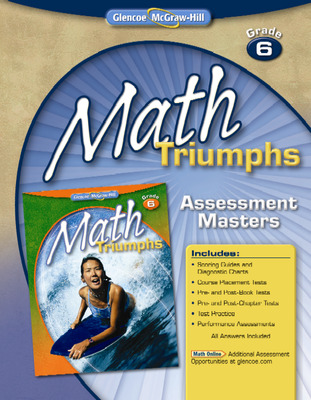Math Triumphs, Grade 6, Assessment Masters
•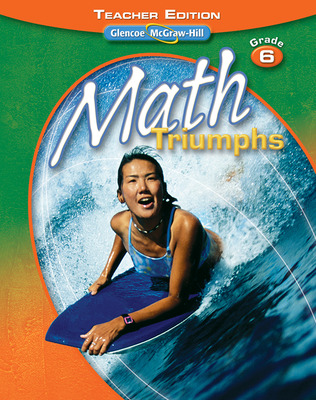Math Triumphs, Grade 6, Teacher Edition
•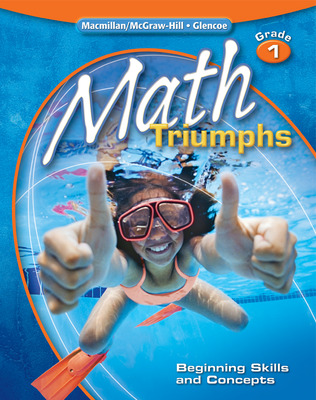Math Triumphs, Grade 1: Beginning Skills and Concepts, Student Study Guide
•Math Triumphs, Grade 2, Student Study Guide, Book 1: Number and Operations
•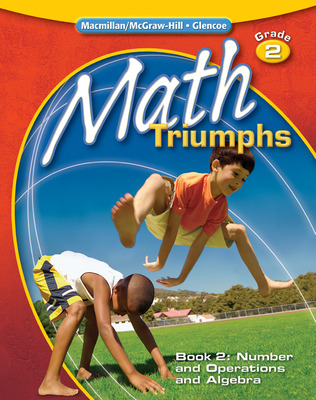Math Triumphs, Grade 2, Student Study Guide, Book 2: Number and Operations and Algebra
•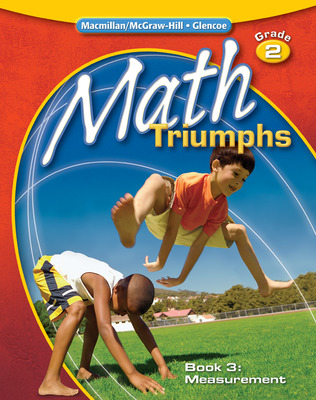Math Triumphs, Grade 2, Student Study Guide, Book 3: Measurement
•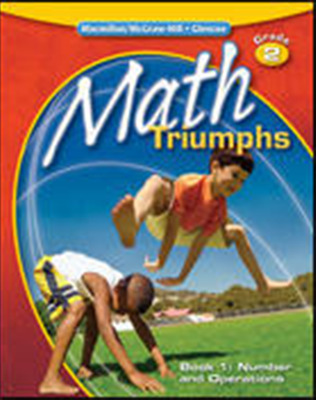Math Triumphs, Grade 2, StudentWorks Plus DVD
•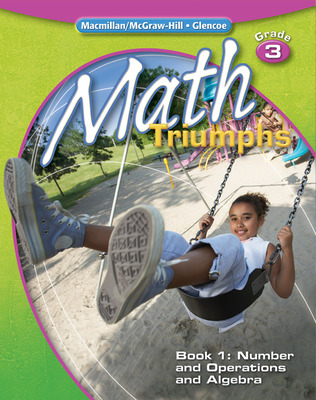Math Triumphs, Grade 3, Student Study Guide, Book 1: Number and Operations and Algebra
•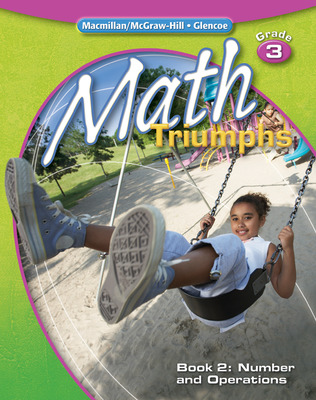Math Triumphs, Grade 3, Student Study Guide, Book 2: Number and Operations
•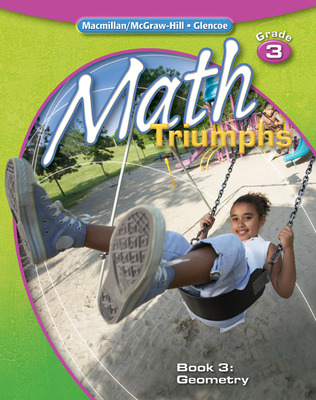Math Triumphs, Grade 3, Student Study Guide, Book 3: Geometry
•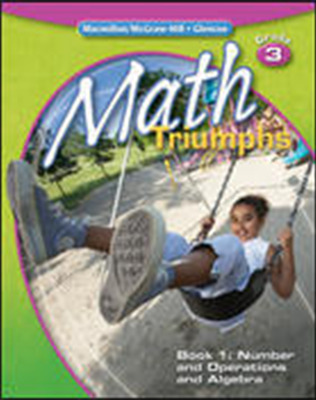Math Triumphs, Grade 3, StudentWorks Plus CD-ROM
•Math Triumphs, Grade 3, StudentWorks Plus DVD
•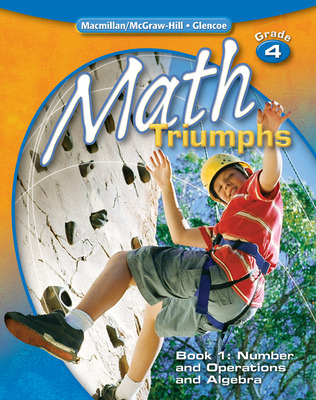Math Triumphs, Grade 4, Student Study Guide, Book 1: Number and Operations and Algebra
•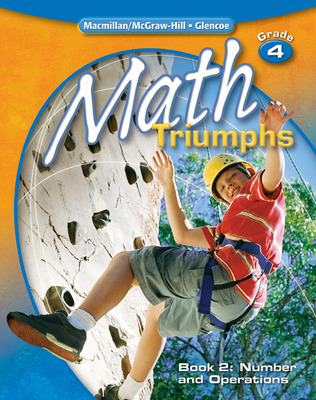Math Triumphs, Grade 4, Student Study Guide, Book 2: Number and Operations
Sort by:

Products

To learn about the specific purchase options for this program, please contact our Customer Service team at 800-338-3987